# RD Sharma Class 8 Solutions Chapter 21 Mensuration II Ex 21.1

## RD Sharma Class 8 Solutions Chapter 21 Mensuration II (Volumes and Surface Areas of a Cubiod and a Cube) Ex 21.1

These Solutions are part of RD Sharma Class 8 Solutions. Here we have given RD Sharma Class 8 Solutions Chapter 21 Mensuration II Ex 21.1

Other Exercises

Question 1.
Find the volume of a cuboid whose
(i) length = 12 cm, breadth = 8 cm, height = 6 cm
(ii) length = 1.2 m, breadth = 30 cm, height = 15 cm
(iii) length = 15 cm, breadth = 2.5 dm, height = 8 cm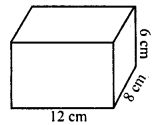Solution:
In a cuboid,
(i) Length (l) = 12 cm
Height (h) = 6 cm
∴ Volume = Ibh = 12 x 8 x 6 cm3 = 576 cm3
(ii) Length (l) = 1.2 m = 120 cm
Height (h) = 15 cm
∴ Volume = Ibh = 120 x 30 x 15 cm3 = 54000 cm3
(iii) Length (l) = 15 cm
Breadth (b) = 2.5 dm = 25 cm
Height (h) = 8 cm
∴ Volume = Ibh
= 15 x 25 x 8 cm3 = 3000 cm2

Question 2.
Find the volume of the cube whose side is
(i) 4 cm
(ii) 8 cm
(iii) 1.5 dm
(iv) 1.2 m
(v) 25 mm.
Solution:
(i) Side of a cube (a) = 4 cm
∴ Volume = a3 = (4)3 cm3 = 4 x 4 x 4 = 64 cm3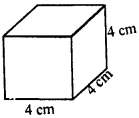(ii) Side of cube (a) = 8 cm
∴ Volume = a3 = (8)3 4 cm
= 8 x 8 x 8 cm3 = 512 cm3
(iii) Side of cube (a) = 1.5 dm = 15 cm
∴ Volume = a3 = (1.5)3 dm2 = (15)3 cm3
= 15 x 15 x 15 = 3375 cm3
(iv) Side of cube (a) = 1.2 m = 120 cm
∴ Volume = a3 = (120)3 cm3
= 120 x 120 x 120 = 1728000 cm3
(v) Side of cube (a) = 25 mm = 2.5 cm.
∴ Volume = a3 = (2.5)3 cm3
= 2.5 x 2.5 x 2.5 cm3 = 15.625 cm3

Question 3.
Find the height of a cuboid of volume 100 cm3 whose length and breadth are 5 cm and 4 cm respectively.
Solution:
Volume of a cuboid =100 cm3
Length (1) = 5 cm
and breadth (b) = 4 cm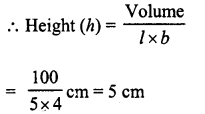Question 4.
A cuboidal vessel is 10 cm long and 5 cm wide, how high it must be made to hold 300 cm3 of a liquid ?
Solution:
Volume of the liquid in the vessel = 300 cm3
Length (l)= 10 cm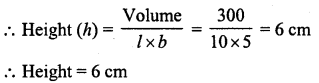Question 5.
A milk container is 8 cm long and 50 cm wide. What should be its height so that it can hold 4 litres of milk ?
Solution:
Capacity of milk = 4 litres
∴ Volume of the container = 4 x 1000 cm3 = 4000 cm3
Length (l) = 8 cm
Width (b) = 50 cm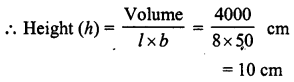Question 6.
A cuboidal wooden block contains 36 cm3 wood. If it be 4 cm long and 3 cm wide, find its height.
Solution:
Volume of wooden cuboid block = 36 cm3
Length (l) = 4 cm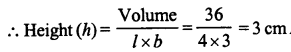Question 7.
What will happen to the volume of a cube, if its edge is (i) halved (ii) trebled ?
Solution:
Let side of original cube = a cm
∴ Volume = a3 cm3
(i) In first case,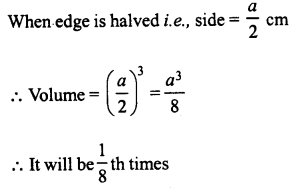(ii) In second case, when side (edge) is trebled, then side = 3a
∴ Volume = (3a)3 = 27a3
∴ It will be 27 times

Question 8.
What will happen to the volume of a cuboid if its (i) Length is doubled, height is same and breadth is halved ? (ii) Length is doubled, height is doubled and breadth is same ?
Solution:
Let l, b and h be the length, breadth and height of the given cuboid respectively.
∴ Volume = lbh.
(i) Length is doubled = 21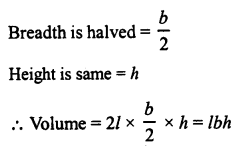∴ The volume will be the same.
(ii) Length is doubled = 21
breadth is same = b height is doubled = 2h
∴ Volume = 2l x b x 2h = 4 lbh
∴ Volume will be 4 times

Question 9.
Three cuboids of dimensions 5 cm x 6 cm x 7 cm, 4 cm x 7 cm * 8 cm and 2 cm x 3 cm x 13 cm are melted and a cube is made. Find the side of cube.
Solution:
Dimensions of first cuboid = 5 cm x 6 cm x 7 cm
∴ Volume = 5 x 6 x 7 = 210 cm3
Dimensions of second cuboid = 4 cm x 7 cm x 8 cm
∴ Volume = 4x 7 x 8 = 224 cm3
Dimensions of third cuboid = 2 cm x 3 cm x 13 cm
∴ Volume = 2 x 3 x 13 = 78 cm3
Total volume of three cubes = 210 + 224 + 78 cm3 = 512 cm3
∴ Volume of cube = 512 cm3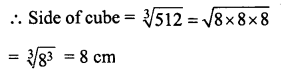Question 10.
Find the weight of solid rectangular iron piece of size 50 cm x 40 cm x 10 cm, if 1 cm3 of iron weighs 8 gm.
Solution:
Dimension of cuboidal iron piece = 50 cm x 40 cm x 10 cm
∴ Volume = 50 x 40 x 10 = 20000 cm3
Weight of 1 cm3 = 8 gm
∴ Total weight of piece = 20000 x 8 gm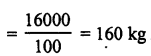Question 11.
How many wooden cubical blocks of side 25 cm can be cut from a log of wood of size 3 m by 75 cm by 50 cm, assuming that there is no wastage ?
Solution:
Length of log (l) = 3 m = 300 cm.
and height (h) = 50 cm
∴ Volume of log = lbh = 300 x 75 x 50 cm3 = 1125000 cm3
Side of cubical block = 25 cm
∴ Volume of one block = a2 = 25 x 25 x 25 cm3 = 15625 cm3
∴ Number of blocks to be cut out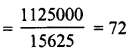Question 12.
A cuboidal block of silver is 9 cm long, 4 cm broad and 3.5 cm in height. From it, beads of volume 1.5 cm2 each are to be made. Find the number of beads that can be made from the block ?
Solution:
Length of block (l) = 9 cm
and height (h) = 3.5 cm
∴ Volume = l x b x h = 9 x 4 x 3.5 cm3 = 126 cm3
Volume of one bead = 1.5 cm3
∴ Number of beads = $$\frac { 126 }{ 105 }$$ = 84

Question 13.
Find the number of cuboidal boxes measuring 2 cm by 3 cm by 10 cm which can be stored in a carton whose dimensions are 40 cm, 36 cm and 24 cm.
Solution:
Length of cuboidal box (l) = 2 cm
and height (h) = 10 cm
∴ Volume = lx b x h = 2 x 3 x 10 = 60 cm3
Volume of carton = 40 x 36 x 24 cm3
= 34560 cm3
∴ Number of boxes to be height in the carton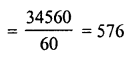Question 14.
A cuboidal block of solid iron has dimensions 50 cm, 45 cm and 34 cm. How many cuboids of size 5 cm by 3 cm by 2 cm can be obtained from the block ? Assume cutting causes no wastage.
Solution:
Dimensions of block = 50 cm, 45 cm, 34 cm
∴ Volume = 50 x 45 x 34 = 76500 cm3
Size of cuboid = 5 cm x 3 cm x 2 cm
∴ Volume of cuboid =  5 x 3 x 2 = 30 cm3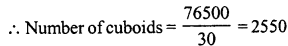Question 15.
A cube A has side thrice as long as that of cube B ? What is the ratio of the volume of cube A to that of cube B ?
Solution:
Let side of cube B = a
Then Volume = a3
and side of cube A = 3a
Volume = (3a)3 = 3a x 3a x 2a = 27a3
∴ Ratio of volume’s A and B = 27a3 : a3
= 27 : 1

Question 16.
An ice-cream brick measures 20 cm by 10 cm by 7 cm. How many such bricks can be stored in a deep fridge whose inner dimensions are 100 cm by 50 cm by 42 cm ?
Solution:
Dimensions of ice cream brick = 20 cm x 10 cm x 7 cm
∴ Volume = 20 x 10 x 7 cm3 = 1400 cm3
Dimensions of inner of fridge = 100 cm x 50 cm x 42 cm = 210000 cm3
∴ Number of bricks to be kept in the fridge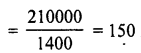Question 17.
Suppose that there are two cubes, having edges 2 cm and 4 cm, respectively. Find the volume V1 and V2 of the cubes and compare them.
Solution:
Side of first cube (a) = 2 cm
∴ Volume (V1) = a3 = (2) = 8 cm3
Similarly side of second cube = 4 cm
and volume (V2) = (4)3 = 64 cm3
Now V2 = 64 cm3 = 8 x 8 cm3
= 8 x V1
⇒ V2 = 8V1

Question 18.
A tea-packet measures 10 cm x 6 cm x 4 cm.How many such tea-packets can be placed in a cardboard box of dimensions 50 cm x 30 cm x 0.2 m ?
Solution:
Dimensions of tea-packet = 10cm x 6cm x 4 cm
∴ Volume =10 x 6 x 4 = 240 cm3
Dimensions of box = 50 cm x 30 cm x 0.2 m
= 50 cm x30 cm x20 cm
∴ Volume = 50 x 30 x 20 = 30000 cm3
∴ Number of tea-packets to be kept = $$\frac { 30000 }{ 240 }$$

Question 19.
The weight of a metal block of size 5 cm by 4 cm by 3 cm is 1 kg. Find the weight of a block of the same metal of size 15 cm by 8 cm by 3 cm.
Solution:
Dimensions of a metal block = 5 cm x 4 cm x 3 cm = 5 x 4 x 3 = 60 cm3
Dimensions of a second block = 15 cm x 8 cm x 3 cm = 15 x 8 x 3 = 360 cm3
But weight of first block = 1 kg
∴ Weight of second block
= $$\frac { 1 }{ 16 }$$ x 360 = 6 kg

Question 20.
How many soap cakes can be placed in a box of size 56 cm x 0.4 m x 0.25 m, it the size of soap cake is 7 cm x 5 cm x 2.5 cm ?
Solution:
Size of box = 56 cm x 0.4 m x 0.25 m = 56 cm x 40 cm x 25 cm
∴ Volume = 56 x 40 x 25 cm3 = 56000 cm3
Size of a soap cake = 7 cm x 5 cm x 2.5 cm
∴ Volume = 7 x 5 x 2.5 cm3 = 87.5 cm3
∴ Number of cakes to be kept in the box
= $$\frac { 56000 }{ 87.5 }$$ = 640

Question 21.
The volume of a cuboid box is 48 cm3. If its height and length are 3 cm and 4 cm respectively, find its breadth.
Solution:
Volume of cuboid box = 48 cm3
Length (l) = 4 cm
Height = (h) = 3 cm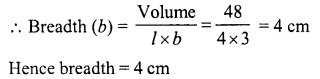Hope given RD Sharma Class 8 Solutions Chapter 21 Mensuration II Ex 21.1 are helpful to complete your math homework.

If you have any doubts, please comment below. Learn Insta try to provide online math tutoring for you.